# Congruent Figures

Congruent figures are geometric figures that have the same shape and size. That is, if you can transform one figure into another figure by a sequence of translations , rotations , and/or reflections , then the two figures are congruent.

If the figures are polygons, then they are congruent if all the corresponding sides and corresponding angles are congruent.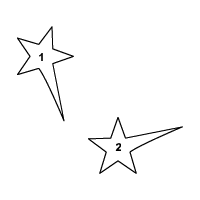Consider the polygon shown.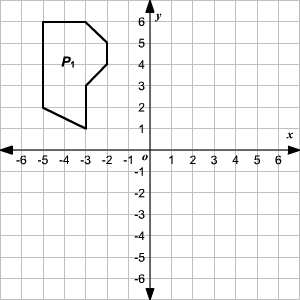Reflect the polygon ${P}_{1}$ about the $y$ -axis.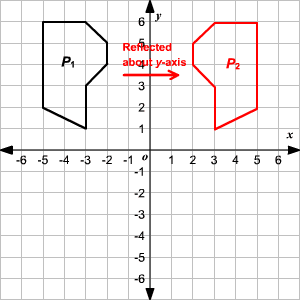The polygons ${P}_{1}$ and ${P}_{2}$ are congruent figures. That is, ${P}_{1}\cong {P}_{2}$ .

Now, rotate the polygon ${P}_{2}$ by $270°$ about the origin.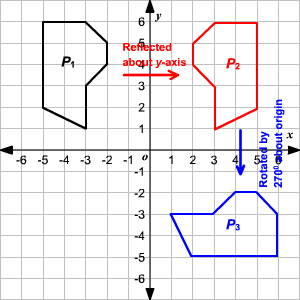Again, ${P}_{2}\cong {P}_{3}$ .

By the transitive property of congruence, ${P}_{1}\cong {P}_{3}$ .

Therefore, the polygon obtained by the transformations is congruent to the initial polygon.

Here, the hexagon $ABCDEF$ is congruent to hexagon $JKLMNO$ .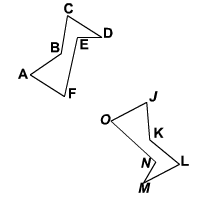Note that the order in which you write the vertices matters; for instance, hexagon $ABCDEF$ is not congruent to hexagon $OJKLMN$ .

Also see congruent triangles .